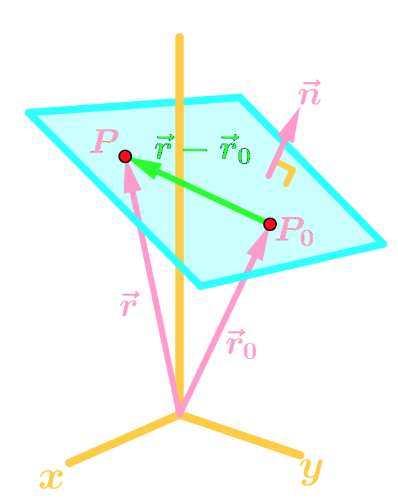# 3-Dimensional planes

### 3-Dimensional planes

#### Lessons

Notes:

Equation of a Plane
Couple sections ago, we saw that the equation of plane can be expressed as $ax+by+cz=d$. However, this equation does not give much information. So suppose we have the following graph:Where $\vec{r}$ and $\vec{r_0}$ are position vectors for points $P$ and $P_0$ respectively, and $\vec{n}$ is a normal vector that is orthogonal (perpendicular) to the plane.
Since $\vec{r} - \vec{r_0}$ is on the plane, then $\vec{n}$ is orthogonal to $\vec{r} - \vec{r_0}$. In other words, their dot products should give 0.
So,

$(\vec{r} - \vec{r_0}) \cdot \vec{n} = 0 \to (\lt x,y,z\gt - \lt x_0,y_0, z_0\gt) \cdot \lt a,b,c\gt = 0$
$\to \lt x-x_0 , y-y_0, z-z_0\gt \cdot \lt a,b,c\gt = 0$
$\to a(x-x_0) + b(y-y_0) + c(z-z_0) = 0$

Which is formula for the equation of a plane.

The key to finding the equation of a plane is finding two things:
1. The normal vector (orthogonal to the plane)
2. A point on the plane.
Then you can just plug those into the formula to get the equation!
• Introduction
3-Dimensional Planes Overview:
a)
Equation of a Plane
• How do we get the formula for the equation?
• $a(x-x_0) + b(y-y_0) + c(z-z_0) = 0$
• What we need for the formula

b)
Finding a Plane with a Parallel Plane & 1 point
• Get the Normal Vector
• Plug into the formula

c)
Finding the Equation of a Plane with 3 points
• Creating 2 vectors
• Using the Cross Product = Normal Vector
• Plug into the formula

• 1.
Finding the Equation of a Plane
Find the equation of the plane which contains the points $(1, 3, 0)$, $(-2, 7, 6)$ and $(1, 0, 1)$.

• 2.
Find the equation of the plane which contains the point $(0, -2, 1)$ and is orthogonal to the line $<1+2t, t, 0>$.

• 3.
Are the Two Planes Parallel, Orthogonal or Neither?
Determine whether the two planes $2x+4y+6z=8$ and $x+2y+3z=1$ are parallel, orthogonal, or neither.

• 4.
Determine whether the two planes $-3x+y+8z=4$ and $2x+6y=1$ are parallel, orthogonal, or neither.

• 5.
Intersection of a Plane and a Line
Determine whether the plane $-3x+5y+z=2$ and line $r(t)=\lt2+3t, -5t, 1-t\gt$ intersect.